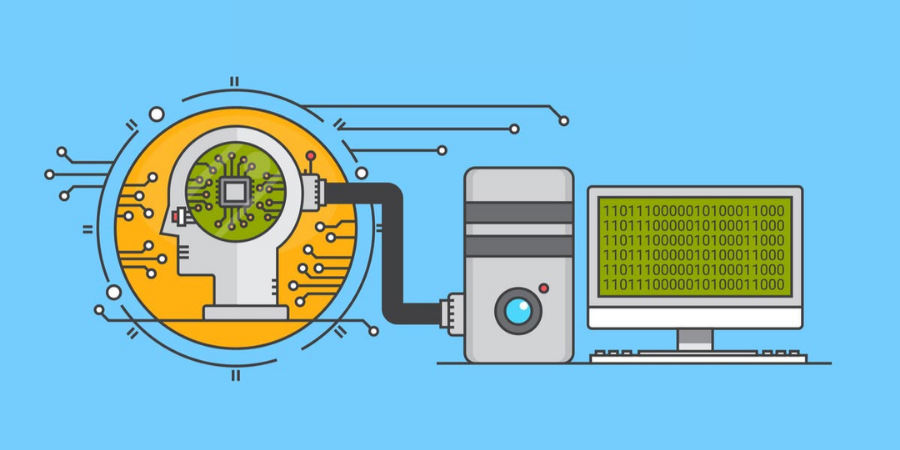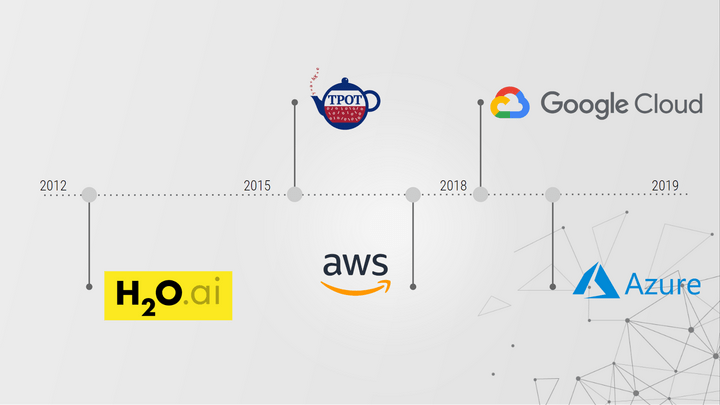# Introduction to Automated Machine LearningS.No Lesson Title
1
2
3
##### Hyperparameter Optimization
3.2 Bayesian Optimization
4
##### Libraries for AutoML
4.1 TPOT
4.2 Hyperopt-Sklearn
4.3 Auto-Keras
5

### Introduction

The idea of training a machine learning model with previous data and then providing it with new data to get predictions sounds very trivial to many of us but in reality that's not the case. There needs to be a highly efficient and good machine learning model pipeline. First of all your model will be good at its predictions when it gets good quality of data to train on, if the data is not pre processed with all the outliers removed, Nan values appropriately filled among some of the tasks then the model will not give expected results. Data pre processing requires some expertise and domain knowledge if you want to obtain a good model.

Apart from data preprocessing the biggest headache is choosing a model from an ocean of models where almost all the models are different with their separate advantages and disadvantages. Even if you narrow it down to a particular set of models the next biggest hurdle is to test them. There are hundreds of combinations of different hyperparameters of models and narrowing down the optimum set of hyperparameters is not a trivial task. Now we have seen that there are a number of different things that have to be looked at before finalizing the machine learning model. Can we make this process simple?

Yes, we can. It can be done with the help of automated machine learning (AutoML). The idea of AutoML is simple and that is to automate as many tasks as possible from start to end of a model building process. This is just a coarser definition of what AutoML models do and in this article we'll dive deeper to see the different AutoML libraries and how they implement the core idea of AutoML. Let's get started.### Why AutoML?

We have already discussed how AutoML can cut down the time taken to build a traditional model from scratch. But there is another interesting thing to notice, in order to cut down the time on traditional model building it does an exhaustive search of possible hyperparameters and models to find the optimum combination. This can take a lot of time and the next thing that comes in mind is if it's worth the time? Yes, it is and following are the three reasons why:

1. If we keep aside deep learning models for a while then other machine learning models can be trained within a reasonable time and with very less computational power. Keep in mind that the manual process of building a model pipeline also takes a lot of time and is not as exhaustive as AutoML solutions.
2. Many people who work as a data scientist have a common script and a set of functions which they commonly use for multiple projects they work on. AutoML is similar to those scripts with the only change being that it doesn't require to the time to built it from scratch.
3. With the advances in cloud computing AutoML can be run in the background over an exhaustive set of machine learning algorithms. This will give a deeper insight about which model work well with the given data and which models don't.

Building machine learning models is an iterative process. You train a model, you get some results out from it and you try to improve the model after seeing how it performed on unseen data. The entire process of building machine learning models can also be said to be experiment drive science and one cannot say if a model will work for sure. That's why when an experiment (model training process) is expensive to run, careful considerations are taken before wasting rescourses on it. Till now we have described AutoML to be an exhaustive way of finding the best possible model and that might have directed some towarda thinking about a simple grid search or random search algorithm which can be very time consuming right? No.

AutoML algorithms do not use the standard gridsearch or randomsearch algorithms but focus on learning from the previous results. In the following section we'll discuss about the techniques used for doing an exhaustive search over different hyperparameters in an optimized manner.

### Hyperparameter Optimization

Before we discuss about hyperparameter optimization techniques let us clarify one thing that there exsits two set of parameters for numerous machine learning models - model parameters and hyperparameters. For example model parameters can be thought of as weights in linear regression or neural networks. They are learned by the model from the data during training. Hyperparameters on the other hand are can be tuned and set by the developer before start of training. They are not dependent on data and are usually constant during training.

Hyperparameter selection is crucial for the performance of the model. The correct set of hyperparamers can be chosen by hand tuning or by running a grid/random search over a set range of hyperparameter values. There are other techniques which can be implemented for this. Following are two of those techniques:

2. Bayesian Optimization

It is a straightforward technique for those who are familiar with gradient descent algorithm. Following are the steps to implement gradient based optimization for AutoML models:

1. Select a sample set of hyperparameters
2. Train a model using these hyperparameters
3. Find the loss of this model using the pre-defined loss function( also called utility score)
4. Find the gradient of this loss function and use it to update the old set of hyperparameters just like the parameters just like the standard gradient descent technique
5. Repeat steps b to d until the loss is below a pre-defined threshold

A few things to keep in mind while using this technique are that the loss function should be differentiable and the gradient values should be scaled with the help of a learning rate parameter to avoid overshooting the optimum value of hyperparameters.

#### Bayesian Optimization

The idea of Bayesian optimization is simple and consists of two parts in parallel - a probabilistic surrogate model and a loss function. The surrogate model has a prior distribution which is assumed to be close to the unknown objective function, On the other hand the loss function/ acquisition function allows us to decide which point/hyperparameter to evaluate on next.

Bayesian optimization takes a point in high dimensional space of hyperparameter configurations, gets the value of loss function for that configuration and then moves on to find a new point to minimize the loss function. If there is no new point that can minimize the loss function more than the last point evaluated then the last point is considered as the optimum set of hyperparameters. Bayesian optimization is designed to ensure that do not spend a lot of resources in hyperparameter space where the loss function values are high and most of the time is spent in the region where loss function values are low. This is possible because whenever we evaluate at a point it is stored.

The performance of this technique depends a lot on choice of correct surrogate model and acquisition function. The traditional surrogate model uses Gaussian process. Other options include random forests or Tree-structured Parzen Estimator (TPE) approaches.

Bayesian optimization is tough to understand for first timers but it is the most commonly used technique in most of the AutoML libraries.

### Libraries for AutoML

There are many tools/libraries which are specifically made for AutoML related tasks. Let's have a look at these tools.

#### TPOT(Tree Based Pipeline Optimization Tool)

It was implemented upon sklearn and is available as a python library. It uses a tree based-structure to represent a model pipeline for a predictive modeling problem which includes tasks such as data preprocessing, modeling algorithms and setting up model hyperparameters. For the following code snippet assume that you have a dataset of features X and a list of labels Y for each sample in X. (We are not sharing the datasets here but the code snippets and results format won't change much for different datasets)

```#install tpot
pip install tpot

# example of tpot for a classification dataset
from sklearn.datasets import make_classification
from sklearn.model_selection import RepeatedStratifiedKFold
from tpot import TPOTClassifier
# define dataset
X, y = make_classification(n_samples=100, n_features=10, n_informative=5, n_redundant=5, random_state=1)
# define model evaluation
cv = RepeatedStratifiedKFold(n_splits=10, n_repeats=3, random_state=1)
# define search
model = TPOTClassifier(generations=5, population_size=50, cv=cv, scoring='accuracy', verbosity=2, random_state=1, n_jobs=-1)
# perform the search
model.fit(X, y)
# export the best model
model.export('tpot_best_model.py')```

Result:

```Generation 1 - Current best internal CV score: 0.9166666666666666
Generation 2 - Current best internal CV score: 0.9166666666666666
Generation 3 - Current best internal CV score: 0.9266666666666666
Generation 4 - Current best internal CV score: 0.9266666666666666
Generation 5 - Current best internal CV score: 0.9266666666666666

Best pipeline: ExtraTreesClassifier(input_matrix, bootstrap=False, criterion=gini, max_features=0.35000000000000003, min_samples_leaf=2, min_samples_split=6, n_estimators=100)
```

#### Hyperopt-Sklearn

Another popular open source python library which implements Bayesian optimization technique. It is designed to optimize models at large scale with hundred of parameters. It also allows the optimization process to distributed across multiple machines and multiple cores. This library use HyperOpt library to describe a search space over possible configurations of Scikit_learn components that also includes preprocessing and classification modules. Now we have some basic knowledge about the library let's look at how to install it and use it (Consider a synthetic dataset with features in X and class labels in Y):

```#install tpot
pip install hyperopt

from sklearn.model_selection import train_test_split
from hpsklearn import HyperoptEstimator
from hpsklearn import any_classifier
from hpsklearn import any_preprocessing
from hyperopt import tpe

# split into train and test sets
X_train, X_test, y_train, y_test = train_test_split(X, y, test_size=0.33, random_state=1)
# define search
model = HyperoptEstimator(classifier=any_classifier('cla'), preprocessing=any_preprocessing('pre'), algo=tpe.suggest, max_evals=50, trial_timeout=30)
# perform the search
model.fit(X_train, y_train)
# summarize performance
acc = model.score(X_test, y_test)
print("Accuracy: %.3f" % acc)
# summarize the best model
print(model.best_model())```

It takes some time for this model to run and the warnings reported can be safely ignored. It prints the accuracy of the best model and the pipeline for later use. The accuracy given is for test dataset.

```Accuracy: 0.848
{'learner': SGDClassifier(alpha=0.0012253733891387925, average=False,
class_weight='balanced', early_stopping=False, epsilon=0.1,
eta0=0.0002555872679483392, fit_intercept=True,
l1_ratio=0.628343459087075, learning_rate='optimal',
loss='perceptron', max_iter=64710625.0, n_iter_no_change=5,
n_jobs=1, penalty='l2', power_t=0.42312829309173644,
random_state=1, shuffle=True, tol=0.0005437535215080966,
validation_fraction=0.1, verbose=False, warm_start=False), 'preprocs': (), 'ex_preprocs': ()}```

#### Auto-Keras

It is used for automating neural network training. The biggest problem faced during automation of neural network training pipeline is neural architecture search (NAS) which is solved by keras to some extent with the help of network morphism and bayesian optimization. There is a detailed blog for this library and you can learn a lot more about the working of this library along with the code snippets on the blog.

Apart from the libraries discussed above many cloud service providers like amazon, google, Microsoft etc. provide support for directly adding AutoML models so that they can be deployed without any hassle. Some of them also have their AutoML modules where you can directly upload the data and deploy a model in a few clicks. We'll not discuss them here as they all have a dedicated user guide on their respective websites.

### Conclusion

As many of you have seen automated machine learning models have the capability of doing a lot of things that usually take a lot of time if done separately. This will raise a doubt in our mind that will they end the role of a data scientist or a machine learning engineer in future? Well, that's not true as a data scientist does many other things like optimizing the entire process which includes selecting the correct algorithm for AutoML models to test on, presenting the insights in a meaning full way and many more. But knowing AutoML techniques is a must nowadays as they have found a wide application. Happy learning!

Send Message
Top